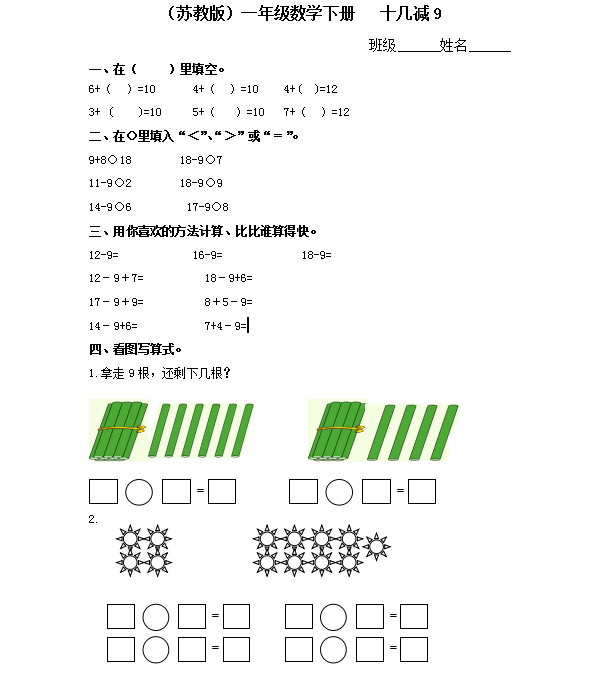（苏教版）小学一年级数学下册 十几减9及答案（5页DOC文档）资料下载

（苏教版）小学一年级数学下册   十几减9

6+（  ）=10      4+（  ）=10    4+(  )=12

3+ (    )=10     5+（   ）=10   7+（  ）=12

9+8○18        18-9○7

11-9○2        18-9○9

14-9○6         17-9○8

12-9=            16-9=             18-9=

12－9＋7=          18－9+6=

17－9＋9=          8＋5－9=

14－9+6=           7+4－9=